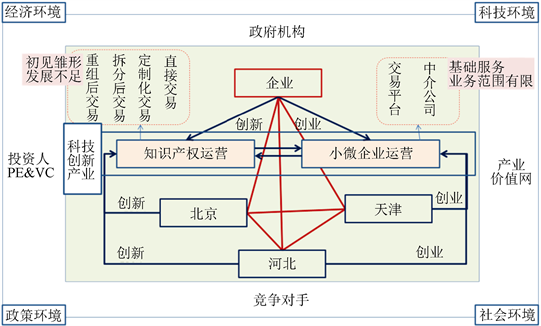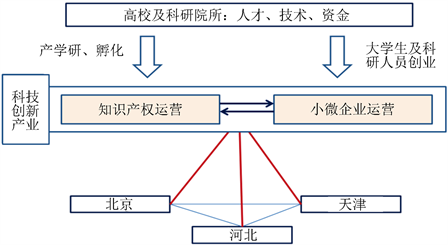京津冀科技协同模式及利益分割初探Science and Technology Cooperative Development Pattern and the Profit Division in Beijing-Tianjin-Hebei Region

• 全文下载: PDF(1636KB)    PP.359-371   DOI: 10.12677/MSE.2018.74043
• 下载量: 162  浏览量: 543   科研立项经费支持

With the cooperative development in Beijing-Tianjin-Hebei region being the national strategy of China, based on the status quo of the sci-tech development, the article is to look for its general co-operative development and provide the basis and references for the cooperative development in Beijing-Tianjin-Hebei region by applying game theory to establish the tangible and intangible profit model of cooperative development.

1. 引言

2. 京津冀科技协同发展模式

“知识运营”既包括知识产权的交易，也包括小微企业的买卖。从人类财富积累角度看，无论是知识产权还是小微企业，都是“知识”的重要表现形式之一。京津冀“知识运营”将京津冀科技创新产业作为整体，将知识产权及小微企业(科技创新载体公司)作为“知识”的表现形式，将“园区、孵化器及产权平台”等作为“知识”的聚集区(包括实体和非实体)、将“PE、VC、人才”等作为“知识”的益生菌，将“金融财税、法律法规、行业协会”等作为“知识”的推进器，将知识的创意、创新、创业、再创新、组合及交易等统一归结为“运营”。京津冀企业视域的“知识运营”模式(图1)，京津冀企业通过创新形成知识产权成果，参与知识产权运营过程，即知识产权的直接交易、多个知识产权重组后交易、知识产权的拆分后交易以及定制化知识产权交易，当前北京在一些行业具备知识产权运营雏形；企业通过内部创业或支持创业参与小微企业运营过程，即小微企业经过交易平台(实体或虚拟)、中介达成交易(或上市)。高校及科研院所视域的京津冀“知识运营”模式(图2)，高校及科研院所是实现教育、科学、技术和产业之间有序推进及相互转化的载体  ，以其自身的知识、技术及专利成为国家知识创新的核心基础；高校教师和科研人员以知识产权人的身份参与直接进行产权交易，或以知识产权作为主体投资企业，主导知识创新的源头；而高校及科研院所的研究员、在校生则以自身的知识储备为基础基于创新链拓展形成众多的创新型小微企业，构成“双创”的主力军。这个过程中，政府并不直接参与到科技协活动中，而是通过发挥其引导作用，将京津冀知识产权交易和小微企业交易纳入到京津冀一体化的总体框架中(图3)，通过制定制度、政策，支持知识产权运营机构及小微企业运营机构为代表的科技创新产业体系的形成。Figure 1. Knowledge operation model from the view of enterprise in Beijing-Tianjin-Hebei regionFigure 2. Knowledge operation model from the view of universities and research institutes in Beijing-Tianjin-Hebei regionFigure 3. Knowledge operation model from the view of government in Beijing-Tianjin-Hebei region

3. 京津冀科技协同发展利益分割机理及分配模型

3.1. 京津冀科技合作利益的内涵Table 1. The profit allocation items of scientific cooperation in Beijing-Tianjin-Hebei region

3.2. 京津冀国际科技协发展利益分配机理

① 有形利益分配问题描述

$V=P-\left(C1+C2\right)$

$V1=tP-C1$

$V2=\left(1-t\right)P-C2$

$C1=C{1}^{0}+{\left(a1b1\right)}^{2}/2$

$C2=C{2}^{0}+{\left(a2b2\right)}^{2}/2$

$P=\frac{{\left(a1d1+a2d2\right)}^{2}}{2}+\left(a1d1+a2d2\right)+{P}^{0}$

$C{1}^{0}$$C{2}^{0}$${P}^{0}$ 为常数，为了确保总收益的收敛性，假设，

$a1\le b1$$a2\le b2$ ，且 $\frac{{\partial }^{2}V}{{\partial }^{2}a1}<0$$\frac{{\partial }^{2}V}{{\partial }^{2}a2}<0$

$V=\frac{{\left(a1d1+a2d2\right)}^{2}}{2}+\left(a1d1+a2d2\right)+{P}^{0}-\left[C{1}^{0}+\frac{{\left(a1b1\right)}^{2}}{2}\right]-\left[C{2}^{0}+\frac{{\left(a2b2\right)}^{2}}{2}\right]$

$V1=t\left[\frac{{\left(a1d1+a2d2\right)}^{2}}{2}+\left(a1d1+a2d2\right)+{P}^{0}\right]-\left[C{1}^{0}+\frac{{\left(a1b1\right)}^{2}}{2}\right]$

$V2=\left(1-t\right)\left[\frac{{\left(a1d1+a2d2\right)}^{2}}{2}+\left(a1d1+a2d2\right)+{P}^{0}\right]-\left[C{2}^{0}+\frac{{\left(a2b2\right)}^{2}}{2}\right]$

② 纳什均衡状态时，成员的努力程度

$\frac{\partial V1}{\partial a1}=t\left[d1\left(a1d1+a2d2\right)+d1\right]-b{1}^{2}=0$

$\frac{\partial V2}{\partial a2}=\left(1-t\right)\left[d2\left(a1d1+a2d2\right)+d2\right]-b{2}^{2}=0$

$a{1}^{*}=\frac{td1{\left(b2\right)}^{2}}{b{1}^{2}b{2}^{2}-td{1}^{2}b{2}^{2}-\left(1-t\right)d{2}^{2}b{1}^{2}}$

$a{2}^{*}=\frac{\left(1-t\right)d2{\left(b1\right)}^{2}}{b{1}^{2}b{2}^{2}-td{1}^{2}b{2}^{2}-\left(1-t\right)d{2}^{2}b{1}^{2}}$

$\frac{a{1}^{*}}{a{2}^{*}}=\frac{t}{1-t}\frac{d1}{d2}\frac{b{2}^{2}}{b{1}^{2}}$

③ 纳什均衡状态，最优分配系数

$\frac{\partial V}{\partial t}=\frac{\partial V}{\partial a{1}^{*}}\frac{\partial a{1}^{*}}{\partial t}+\frac{\partial V}{\partial a{2}^{*}}\frac{\partial a{2}^{*}}{\partial t}$

$\frac{\partial a{1}^{*}}{\partial t}=\frac{d1b{2}^{2}\left(b{2}^{2}b{1}^{2}-d{2}^{2}b{1}^{2}\right)}{{\left[\left(b{2}^{2}b{1}^{2}-d{2}^{2}b{1}^{2}\right)+t\left(d{2}^{2}b{1}^{2}-d{1}^{2}b{2}^{2}\right)\right]}^{2}}$

$\frac{\partial a{2}^{*}}{\partial t}=\frac{-d2b{1}^{2}\left(b{2}^{2}b{1}^{2}-d{1}^{2}b{2}^{2}\right)}{{\left[\left(b{2}^{2}b{1}^{2}-d{2}^{2}b{1}^{2}\right)+t\left(d{2}^{2}b{1}^{2}-d{1}^{2}b{2}^{2}\right)\right]}^{2}}$

V对 $a{1}^{*},a{2}^{*}$ 求偏导得：

$\frac{\partial V}{\partial a{1}^{*}}=\left(d1a{1}^{*}+d2a{2}^{*}\right)d1+d1-b{1}^{2}$

$\frac{\partial V}{\partial a{2}^{*}}=\left(d1a{1}^{*}+d2a{2}^{*}\right)d2+d2-b{2}^{2}$

$\frac{\partial V}{\partial a{1}^{*}}=\frac{1-t}{t}b{1}^{2}a{1}^{*}$

$\frac{\partial V}{\partial a{2}^{*}}=\frac{d2}{d1}b{1}^{2}a{1}^{*}$

$\frac{\partial V}{\partial t}=\frac{b{1}^{2}\left[\frac{t}{1-t}d1b{2}^{2}\left(b{1}^{2}b{2}^{2}-d{2}^{2}b{1}^{2}\right)-\frac{d{2}^{2}b{1}^{2}}{d1}\left(b{1}^{2}b{2}^{2}-d{1}^{2}b{2}^{2}\right)\right]}{{\left[\left(b{1}^{2}b{2}^{2}-d{2}^{2}b{1}^{2}\right)-t\left(d{2}^{2}b{1}^{2}-d{1}^{2}b{2}^{2}\right)\right]}^{2}}$

$\frac{\partial V}{\partial t}=0$ ，得：

$\frac{t}{1-t}d1b{2}^{2}\left(b{1}^{2}b{2}^{2}-d{2}^{2}b{1}^{2}\right)-\frac{d{2}^{2}b{1}^{2}}{d1}\left(b{1}^{2}b{2}^{2}-d{1}^{2}b{2}^{2}\right)=0$

${t}^{*}=\frac{d{1}^{2}\left(b{2}^{2}-d{2}^{2}\right)}{d{1}^{2}\left(b{2}^{2}-d{2}^{2}\right)+d{2}^{2}\left(b{1}^{2}-d{1}^{2}\right)}$

${R}_{a}^{0}>\frac{{R}_{a}^{*}}{{R}_{a}^{*}+{R}_{b}^{*}}\left({R}_{a}^{0}+{R}_{b}^{0}\right)$

${R}_{b}^{0}>\frac{{R}_{b}^{*}}{{R}_{a}^{*}+{R}_{b}^{*}}\left({R}_{a}^{0}+{R}_{b}^{0}\right)$

$\frac{{R}_{a}^{*}}{{R}_{a}^{*}+{R}_{b}^{*}}\left({R}_{a}^{1}+{R}_{b}^{1}\right)<{R}_{a}^{1}<\frac{{R}_{a}^{0}}{{R}_{a}^{0}+{R}_{b}^{0}}\left({R}_{a}^{1}+{R}_{b}^{1}\right)$

$\frac{{R}_{b}^{0}}{{R}_{a}^{0}+{R}_{b}^{0}}\left({R}_{a}^{1}+{R}_{b}^{1}\right)<{R}_{b}^{1}<\frac{{R}_{b}^{*}}{{R}_{a}^{*}+{R}_{b}^{*}}\left({R}_{a}^{1}+{R}_{b}^{1}\right)$

$\frac{{R}_{a}^{tn}}{{R}_{a}^{tn}+{R}_{b}^{tn}}=\frac{{R}_{a}^{*}}{{R}_{a}^{*}+{R}_{b}^{*}}$

$\frac{{R}_{b}^{tn}}{{R}_{a}^{tn}+{R}_{b}^{tn}}=\frac{{R}_{b}^{*}}{{R}_{a}^{*}+{R}_{b}^{*}}$

3.3. 京津冀国际科技协同发展利益分配模型

${\omega }_{ij}=\frac{{C}_{ij}{\left(1+r\right)}^{m+1-j}{P}_{ioj}}{{\sum }_{i=1}^{n}{C}_{ij}{\left(1+r\right)}^{m+1-j}{P}_{ioj}}$

${\Phi }_{ij}=\frac{{C}_{ij}{\left(1+r\right)}^{m+1-j}{P}_{ioj}}{{\sum }_{i=1}^{n}{C}_{ij}{\left(1+r\right)}^{m+1-j}{P}_{ioj}}V\left(N\right)$Table 2. Integrated rules and indicators of tangible benefit allocation

${R}_{ij}=1-\left(1-{R}_{\left(a\right)ij}\right)\left(1-{R}_{\left(b\right)ij}\right)\left(1-{R}_{\left(c\right)ij}\right)$

${v}_{ij}=\frac{{R}_{ij}}{{\sum }_{i=1}^{n}\text{ }{R}_{ij}}$

${{\Phi }^{″}}_{ij}=\frac{{R}_{ij}}{{\sum }_{i=1}^{n}{R}_{ij}}V\left(N\right)$Table 3. Risk-based rules and indicators of tangible benefit allocation

${h}_{i}\left[z\left(x\right),\text{\hspace{0.17em}}\text{\hspace{0.17em}}0<{h}_{i}<1,\text{\hspace{0.17em}}\text{\hspace{0.17em}}\underset{i=1}{\overset{n}{\sum }}{h}_{i}=1,\text{\hspace{0.17em}}\text{\hspace{0.17em}}\frac{\partial {k}_{i}}{\partial {h}_{i}}>0$

$\underset{{h}_{i}\to 0}{\mathrm{lim}}{u}_{i}\left({k}_{i}\right)={u}_{i}\left({g}_{i}\right)=0,\text{\hspace{0.17em}}\text{\hspace{0.17em}}\text{\hspace{0.17em}}\underset{{h}_{i}\to 1}{\mathrm{lim}}{u}_{i}\left({k}_{i}\right)={u}_{i}\left(K\right)$

${h}_{i}^{t}={\pi }_{i,1}{l}_{i,1}^{t}+{\pi }_{i,2}{l}_{i,2}^{t}+{\pi }_{i,3}{l}_{i,3}^{t}+\cdots +{\pi }_{i,m}{l}_{i,m}^{t}$

${\pi }_{i,1}>{\pi }_{i,2}>{\pi }_{i,m},\text{\hspace{0.17em}}\text{\hspace{0.17em}}且0<{\pi }_{i,m}<0,\text{\hspace{0.17em}}\text{\hspace{0.17em}}\underset{p=1}{\overset{m}{\sum }}{\pi }_{i,p}=1$

$\underset{{r}_{i}\to 0}{\mathrm{lim}}\left(\frac{{s}_{i}}{{r}_{i}}\right),\text{\hspace{0.17em}}且\underset{{r}_{i}\to 0}{\mathrm{lim}}\left(\frac{{s}_{i}}{{r}_{i}}\right)=\frac{{u}_{i}\left({k}_{i}\right)}{{{u}^{\prime }}_{i}\left(ki\right)}$

${q}_{i}=\frac{{u}_{i}\left({k}_{i}\right)}{{{u}^{\prime }}_{i}\left(ki\right)}$

$\frac{\text{d}{q}_{i}}{\text{d}{k}_{i}}=\frac{{\left[{{u}^{\prime }}_{i}\left({k}_{i}\right)\right]}^{2}-{u}_{i}\left({k}_{i}\right){{u}^{″}}_{i}\left({k}_{i}\right)}{{\left[{{u}^{\prime }}_{i}\left({k}_{i}\right)\right]}^{2}}>0$

$\mathrm{max}\underset{i=1}{\overset{2}{\prod }}{u}_{i}^{{h}_{i}\left(t\right)}$

$\frac{{k}_{i}\left(t\right)}{{\sum }_{i=1}^{2}\text{ }\text{ }\text{ }{k}_{i}\left(t\right)}=\frac{{k}_{i}^{*}}{{\sum }_{i=1}^{2}\text{ }\text{ }{k}_{i}^{*}}$

$\mathrm{max}{\left[{u}_{1}\left(t\right)-{d}_{1}\left(t\right)\right]}^{{h}_{1}\left(t\right)}{\left[{u}_{2}\left(t\right)-{d}_{2}\left(t\right)\right]}^{{h}_{2}\left(t\right)}$

$\frac{{h}_{1}}{{h}_{2}}=\frac{{q}_{1}}{{q}_{2}}$

$u=\mathrm{max}\left[{u}_{1}{\left(t\right)}^{{h}_{1}\left(t\right)}×{u}_{2}{\left(t\right)}^{{h}_{2}\left(t\right)}×{u}_{3}{\left(t\right)}^{{h}_{3}\left(t\right)}×\cdots ×{u}_{n}{\left(t\right)}^{{h}_{n}\left(t\right)}\right]=\mathrm{max}\underset{i=1}{\overset{n}{\prod }}{u}_{i}{\left(t\right)}^{{h}_{i}\left(t\right)}$

$\frac{{h}_{i}}{{h}_{j}}=\frac{{q}_{i}}{{q}_{j}},\text{\hspace{0.17em}}\text{\hspace{0.17em}}i,j=1,2,\cdots ,n且i\ne j$

${u}_{i}{\left(t\right)}^{*}×{u}_{j}{\left(t\right)}^{*}=\frac{{h}_{i}\left(t\right)}{{h}_{j}\left(t\right)}×\frac{{u}_{i}{\left(t\right)}^{\prime }}{{u}_{j}{\left(t\right)}^{\prime }}$

${u}_{i}{\left(t\right)}^{*}$ 即为成员i在最优解处获得的效用目标，协同创新成员效用大小对比取决于他们的谈判力大小和取得最优解时效用评价值大小的对比。最优解处的边际值给定时，某方的谈判力越大，最终达成效用目标的可能性也就越大；当最优解处的谈判力一定时，效用函数的具体结构和形式也就决定了最优解处效用目标的达成程度。

4. 构建促进京津冀科技协同发展的政府保障体系

4.1. 基础设施共建共享

4.2. 创新网络共建共享

4.3. 建立协同发展管理机制

1) 制定协同发展战略，完善政策保障体系

2) 成立3 + 1协调机构，建立京津冀多边谈判制度

3) 打造科技合作平台，发挥行业协会及非盈利组织的作用

  魏后凯, 等. 中国区域协调发展研究[M]. 北京: 中国社会科学出版社, 2012: 20.  范恒山, 孙久文, 陈宣庆, 等. 中国区域协调发展研究[M]. 北京: 商务印书馆, 2012: 14-15.  祝尔娟, 邬晓霞. 推进京津冀区域经济一体化[J]. 经济学动态, 2012(2): 156-158.  孙久文, 丁鸿君. 京津冀区域经济一体化进程研究[J]. 经济与管理研究, 2012(7): 52-57.  窦文章, 宋丹, 赵玲玲. 科技创新产业的区域迭代[M]. 北京: 中国科学技术出版社, 2017.  文魁, 祝尔娟. 首都圈发展报告(2013)——承载力测度与对策[M]. 北京: 社会科学文献出出版社, 2013: 367-380.  窦文章, 等. 创新策略与产权盈利的双重博弈[M]. 北京: 知识产权出版社, 2011.  罗利, 鲁若愚. 产学研合作对策模型[J]. 管理工程学报, 2000, 14(1): 1-4.  刘新元. 利益冲突和制度变迁[M]. 武汉: 武汉大学出版社, 2002.  刘学, 庄乾志. 合作创新的风险分摊与利益分配[J]. 科研管理, 1998, 19(15): 31-35.  Lemaire, J. (1991) Cooperative Game Theory and Its Insurance Application. Astin Bulletin, 21, 17-40. https://doi.org/10.2143/AST.21.1.2005399  洪军, 陈森发. 价值网中主体动态博弈分析[J]. 管理工程学报, 2004, 18(4): 127-130.  Jia, N.X. and Yokoyama, R. (2003) Profit Allocation of Independent Power Producers Based on Cooperative Game Theory. Electrical Power and Energy Systems, 25, 633-641. https://doi.org/10.1016/S0142-0615(02)00180-1  Flame, S.D. and Jourani, A. (2003) Strategic Behavior and Partial Cost sharing. Games and Economic Behavior, 43, 44-56. https://doi.org/10.1016/S0899-8256(02)00547-X  Krus, L. and Bronisz, P. (2000) Cooperative Game Solution Concepts to a Cost Allocation Problem. European Journal of Operational Research, 122, 258-271. https://doi.org/10.1016/S0377-2217(99)00232-5  申学武, 聂规划, 沈凌. 科技型动态联盟中知识共享的激励机制与对策研究[J]. 科学学与科学技术管理, 2007, 28(6): 92-95.  田肇云, 葛新权. 动态联盟知识共享与合作的决策分析[J]. 工业技术经济, 2007, 26(4): 104-105.  戴建华, 薛恒新. 基于Shapley值法的动态联盟伙伴企业利益分配策略[J]. 中国管理科学, 2004, 12(4): 33-36.